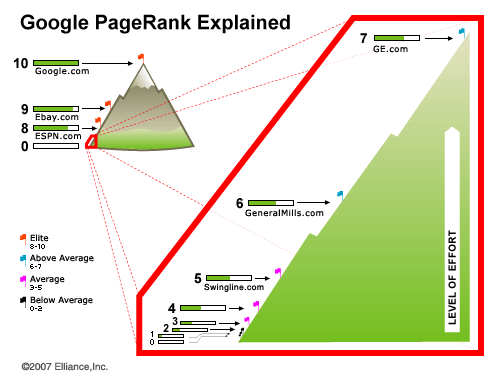200+国企、上市企业的选择

1. 提供有吸引力、有价值的网站内容，这样站长们会主动和你进行友情链接，从而提高你的外部链接值。
3. 可将网站添加到行业门户站点、网上论坛、留言簿等等各种允许添加网址链接的地方。
4. 与其他网站交换链接来提高链接权值。

PR(A) = (1-d)+ d(PR(t1)/C(t1)+ ... + PR(tn)/C(tn))

PR(A) = (1-0.85) + 0.85*(4/1)
=0.15+0.85*4=0.15+3.40=3.55

PR(A) = (1-0.85)+ 0.85*(4/2)
=0.15+0.85*2=0.15+1.70=1.85

PR(A) = (1-0.85) + 0.85*(0/N) + 0.85*(0/N) + ... + 0.85*(0/N)
=0.15+0=0.15

PR值分布图解：1、观察并掌握谷歌PR值的更新规律对于有效提升网站PR值有很大的辅助作用。

2、网站快速提高PR值的方法屡见不鲜，最重要的是准确计算更新周期为下次更新做好准备。

3、新站应该先以基链为跳台防止被K，在下次数据更新前导入大量高质量首页单向链接适可而止。

4、培养小范围局部“链轮”防止过度优化，可以有效增加目标网站权重传递，在操作难度上较难把握。

5、注重相关性链接数量导入之后，制造同等量的广泛性链接，防止搜索引擎误判为作弊嫌疑而被和谐打击。

6、没有资源并不足为虑，没有资金拿什么来购入资源？适量的买入高质量的友情链接，打一炮换一个地方，并再来一次。

Tags：